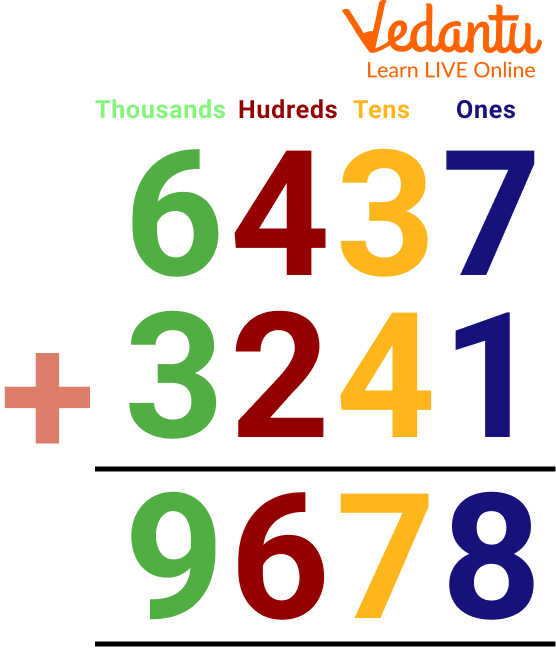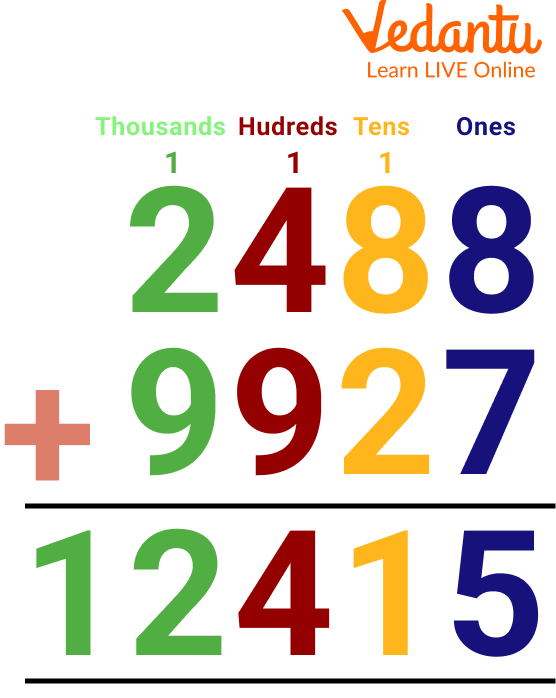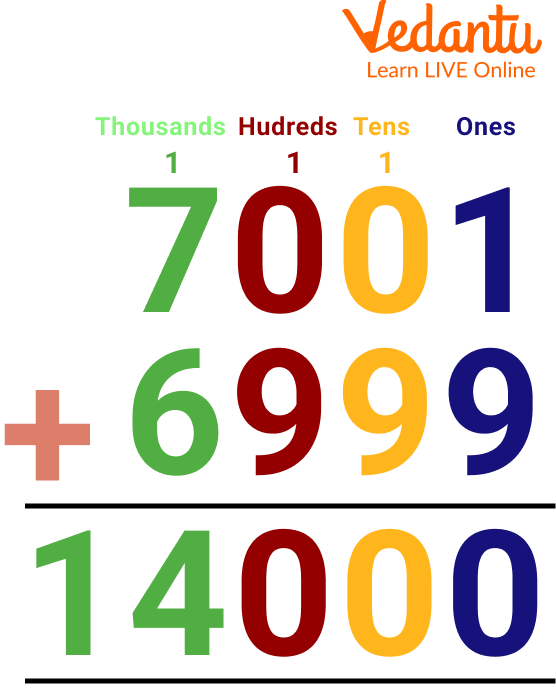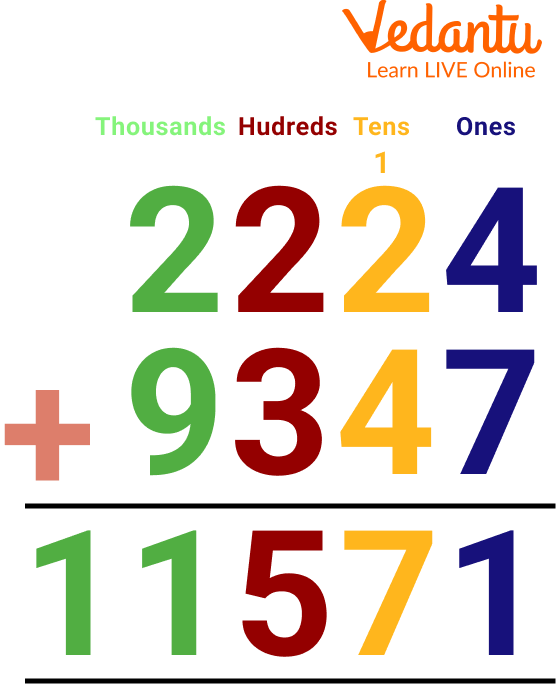Courses
Courses for Kids
Free study material
Free LIVE classes
MoreLIVE
Join Vedantu’s FREE Mastercalss

The addition is one of the basic mathematical operations which is a process of adding two or more two quantities or numbers together to get the total. The numbers which are added to each other are called addends and the result of the total that we get after doing the operation is called the sum. The easiest way to get the sum of 4 digit numbers is to first arrange them according to their place values: ones of the number in the one's place, tens in the tens place, hundreds in the hundreds place, and thousands in the thousands place.

## Steps to Solve the 4 Digit Addition

Once you place your numbers according to their place values, next is to add all the numbers which lie to their ones, tens, hundreds, and thousands. Keep in mind that it's always important to add the ones first and then the rest.

When we do the addition of numbers, always start adding the numbers from the right-hand side to the left-hand side.

In short, the rules are the same as we do for the numbers which are less than 4 digits or so.

For example: (i) 6437 + 3241

Step 1: Adding the numbers which fall to their ones face value => 7+1=8

Step 2: Adding the numbers which fall to their tens face value => 3+4=7

Step 3: Adding the numbers which fall to their hundreds of face value => 4+2=6

Step 4: Adding the numbers which fall to their thousands of face value => 6+3=9Arrangement of Numbers According to their Face Values for Addition

Example: (ii) 2488 + 9927Adding the ones 8+7=15, carry forward 1, and write the 5 in the one's column

Adding the tens 1+8+2= 11, carry forward and write the 1 in the tens column

Adding the hundreds 1+3+9=13, carry forward 1, and write 3 in the hundreds column

Adding the thousands 1+2+9= 12, write 12 as it is.

The sum of 2388 + 9927 is 12315.

NOTE: If the sum of numbers has a double-digit, then you carry it onto the next digit except for the last digit because you can directly write it down in the answer’s place. No need to carry.

## Solved Examples

Q 1. 7001 + 6999Solved Example of 4-Digit Addition Question

Ans: Adding the ones 1+9=10, carry forward 1, and write the 0 in the one's column

Adding the tens 1+0+9= 10, carry forward and write the 0 in the tens column

Adding the hundreds 1+0+9=10, carry forward 1, and write 0 in the hundreds column

Adding the thousands 1+7+6= 14, write 14 as it is.

The sum of 7001 + 6999 is 14000.

Q 2. 2224 + 9347Solved Example of 4-Digit Addition Question

Ans: Adding the ones 4+7=11, carry forward 1, and write the 1 in the one's column

Adding the thousands 2+9= 11, write 11 as it is.

The sum of 2224 + 9347 is 11571.

Q 1. 4994 + 3445

Ans: 8439

Q 2. 9543 + 3543

Ans: 13086

Q 3. 2998 + 6758

Ans: 9756

Q 4. 5379 + 3909

Ans: 9288

Q 5. 1209 + 6480

Ans: 7689

Q 6. 7645 + 6598

Ans: 14,243

Q 7. 5347 + 4650

Ans: 9,997

Q 8. 8905 + 5321

Ans: 14,226

## Summary

To sum up two or more numbers, the addition operation and its process is performed and this article has tried to teach the easiest way to add 4 digit numbers. You have looked through the stepwise procedure of how to add 4 digit numbers and a few solved examples have also been shown in the article for better understanding. Addition sums of 4 digits are done with regrouping i.e. solving the sums by placing them in vertical columns. For better understanding, solve a maths 4-digit addition worksheet.

Last updated date: 21st Sep 2023
Total views: 108.9k
Views today: 3.08k

## FAQs on 4 Digit Addition

1. How many 4-digit numbers are there?

The smallest 4-digit number is 1000 and the greatest 4-digit number is 9999. There are 9000 four-digit numbers in all.

2. What is Commutative law?

According to the commutative law of addition, when two numbers are added, the result is equal to the addition of their interchanged positions.

A + B = B + A

Examples:

1 + 2 = 2 + 1 = 3

4 + 5 = 5 + 4 = 9

3. How is the 4-digit addition with regrouping done?

4-digit addition with regrouping is done by placing the values in vertical columns according to their place values. In this method, we start adding the values of the one and move to the left. One digit will be added with the one digit of another number, a tens digit will be added with the tens digit of another number, and hundreds and thousands of digits will be added to the hundreds and thousands digit of another number respectively. Regrouping can also be understood by the term ‘carrying over. Addition with regrouping can be practiced by attempting a maths 4-digit addition worksheet.